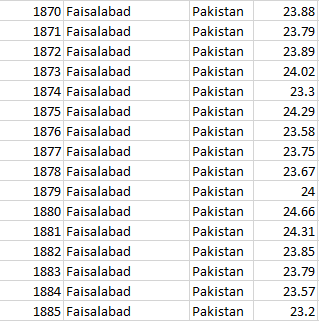# Little Help

here is the problem i can’t solve

1. Output a table that has the overall average of each country(Done)
2. Classify the countries Temperature into “Low/Mid/High” ( EXAMPLE : IF the max temp is 90 and the min temp is 0 then from 0 to 30 is “Low”, from 30 to 60 “Mid”, from 60 to 90 “High” ).(Done)
3. ``````  Output a table that has the difference between the average of the country in each year and the average global temp in the last 22 years.[have problem]
``````

Can’t understand how to calculate the difference from two different csv files
any helpHello @modyali2 ,

welcome to the forum.

Let me understand: to compute point 3, you need two things.

• The first one is the average for a certain country, and you have calculated it in point 1.
• The second one is a fixed value, represented by the global temperature of the last 22 years. I assume you have data to calculate it. Use a Table Row to Variable to create a variable with its value.

Then, if you have both, take your results of point 1 and use a Column Expression node to compute the difference between the column with the country values and the variable with the global average.

Tell me if something is unclear or if I misunderstood something.

Have a nice day,
RB

1 Like

Thank you for your support but i already figured it out only one point to achieve to get finished i can’t draw a histogram for tempreature values but iam still studing

1 Like

This topic was automatically closed 90 days after the last reply. New replies are no longer allowed.